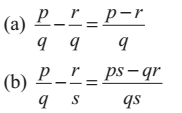# Fundamental Operations on Rational Numbers

### 1. Addition of Rational Numbers

(a) Consider the addition of rational numbers with same denominators: p/q and r/q

p/q + r/q = (p + r)/q

The addition of two rational numbers with common denominator is the rational number with common denominator and numerator as the sum of the numerators of the two rational numbers.

For example, 2/3 + 5/3 = (2+5)/3 = 7/3

(b) Consider the two rational numbers with different denominators: p/q and r/s

p/q + r/s = ps/qs + rq/sq = (ps + rq)/qs

The sum of two rational numbers with different denominators is a rational number with the denominator equal to the product of the denominators of two rational numbers and the numerator equal to sum of the product of the numerator of first rational number with the denominator of second and the product of numerator of second rational number and the denominator of the first rational number.

### 2. Subtraction of Rational Numbers

The subtraction is similar to addition.### 3. Multiplication of Rational Numbers

Multiplication of two rational number p/q and r/s, q ≠ 0, s ≠ 0 is the rational number pr/ps, where qs ≠ 0.

### 4. Division of Rational Numbers

Division of two rational numbers p/q and r/s, such that q ≠ 0, s ≠ 0, is the rational number ps/qr, where qr ≠ 0.